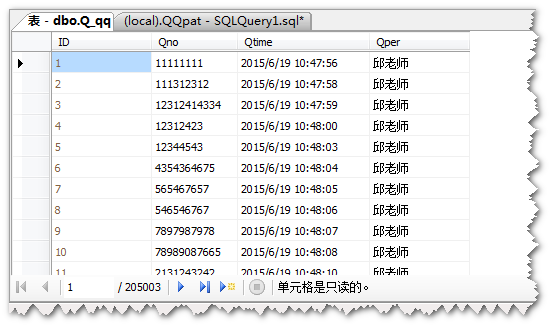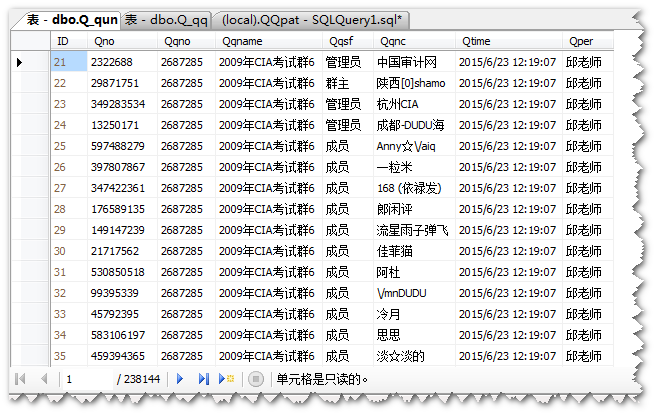# SQL查重并删除电脑cpu直达100%

``delete from Q_qq where ID not in(select min(ID) from Q_qq group by Qno having count(Qno)>=1)````delete tmp from( select row_num = row_number() over(partition by Qno,Qqno order by Qtime asc) from Q_qun where Qtime>getdate()-1) tmp where row_num > 1 ``...全文
76 点赞 收藏 4

4 条回复

``````delete from Q_qq
where EXISTS (SELECT *
FROM Q_qq t
WHERE t.Qno = Q_qq.Qno
AND t.ID < Q_qq.ID)``````

Tiger_Zhao 2015-09-18

``````delete from Q_qq
where EXISTS (SELECT *
FROM Q_qq t
WHERE t.Qno = Q_qq.Qno
AND t.ID < Q_qq.ID)``````

2.1w+

MS-SQL Server 疑难问题